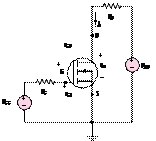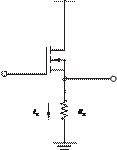### Create an Account

Home / Questions / The circuit of Figure P1118 is called a source follower and acts as a voltage controlled ...

# The circuit of Figure P1118 is called a source follower and acts as a voltage controlled current source VCCS Drain source voltage

The circuit of Figure P11.18 is called a source follower, and acts as a voltage-controlled current source (VCCS).

Drain-source voltage vDS, V

(a)12 VVG

VL

Figure P11.13

(b)

Figure P11.18

a.    Determine IL  if VG  = 10 V, RL  = 2 s-2K =

0.5 A/V2, VT  = 4 V.

b.    If the power rating of the MOSFET is 50 W, how small can Rbe?

Jun 18 2020 View more View LessSubscribe To Get Solution